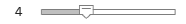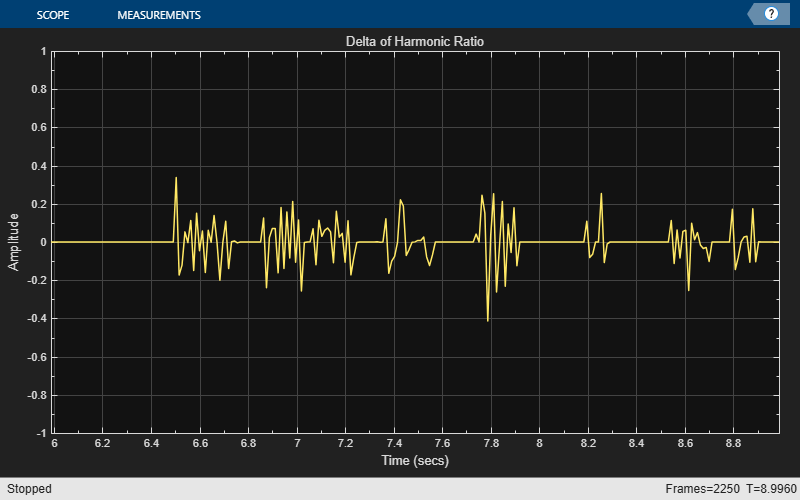Main Content

# audioDelta

Compute delta features

## Syntax

``delta = audioDelta(x)``
``delta = audioDelta(x,deltaWindowLength)``
``delta = audioDelta(x,deltaWindowLength,initialCondition)``
``[delta,finalCondition] = audioDelta(x,___)``

## Description

example

````delta = audioDelta(x)` returns the delta features of input `x`. Columns of `x` are treated as independent channels.```

example

````delta = audioDelta(x,deltaWindowLength)` specifies the delta window length.```

example

````delta = audioDelta(x,deltaWindowLength,initialCondition)` specifies the initial condition of the filter.```

example

````[delta,finalCondition] = audioDelta(x,___)` also returns the final condition of the filter.```

## Examples

collapse all

Read in an audio file.

`[audioIn,fs] = audioread('Counting-16-44p1-mono-15secs.wav');`

Create an `audioFeatureExtractor` object to extract some spectral features over time from the audio. Call `extract` to extract the audio features.

```afe = audioFeatureExtractor('SampleRate',fs, ... 'spectralCentroid',true, ... 'spectralSlope',true); audioFeatures = extract(afe,audioIn);```

Call `audioDelta` to approximate the first derivative of the spectral features over time.

`deltaAudioFeatures = audioDelta(audioFeatures);`

Plot the spectral features and the delta of the spectral features.

```map = info(afe); tiledlayout(2,1) nexttile plot(audioFeatures(:,map.spectralCentroid)) ylabel('Spectral Centroid') nexttile plot(deltaAudioFeatures(:,map.spectralCentroid)) ylabel('Delta Spectral Centroid') xlabel('Frame')``````tiledlayout(2,1) nexttile plot(audioFeatures(:,map.spectralSlope)) ylabel('Spectral Slope') nexttile plot(deltaAudioFeatures(:,map.spectralSlope)) ylabel('Delta Spectral Slope') xlabel('Frame')```The delta and delta-delta of mel frequency cepstral coefficients (MFCC) are often used with the MFCC for machine learning and deep learning applications.

Read in an audio file.

`[audioIn,fs] = audioread("Counting-16-44p1-mono-15secs.wav");`

Use the `designAuditoryFilterBank` function to design a one-sided frequency-domain mel filter bank.

```analysisWindowLength = round(fs*0.03); fb = designAuditoryFilterBank(fs,"FFTLength",analysisWindowLength);```

Use the `stft` function to convert the audio signal to a complex, one-sided frequency-domain representation. Convert the STFT to magnitude and apply the frequency-domain filtering.

```[S,~,t] = stft(audioIn,fs,"Window",hann(analysisWindowLength,"periodic"),"FrequencyRange","onesided"); auditorySTFT = fb*abs(S);```

Call the `cepstralCoefficients` function to extract the MFCC.

`melcc = cepstralCoefficients(auditorySTFT);`

Call the `audioDelta` function to compute the delta MFCC. Call `audioDelta` again to compute the delta-delta MFCC. Plot the results.

```deltaWindowLength =21; melccDelta = audioDelta(melcc,deltaWindowLength); melccDeltaDelta = audioDelta(melccDelta,deltaWindowLength); coefficientToDisplay =4; tiledlayout(3,1) nexttile plot(t,melcc(:,coefficientToDisplay+1)) ylabel('Coefficient ' + string(coefficientToDisplay)) nexttile plot(t,melccDelta(:,coefficientToDisplay+1)) ylabel('Delta') nexttile plot(t,melccDeltaDelta(:,coefficientToDisplay+1)) xlabel('Time (s)') ylabel('Delta-Delta')```You can calculate the delta of streaming signals by passing state in and out of the `audioDelta` function.

Create a `dsp.AudioFileReader` object to read an audio file frame-by-frame. Create an `audioDeviceWriter` object to write audio to your speaker. Create a `timescope` object to visualize the change in harmonic ratio over time.

```fileReader = dsp.AudioFileReader("FemaleSpeech-16-8-mono-3secs.wav","SamplesPerFrame",32,"PlayCount",3); deviceWriter = audioDeviceWriter("SampleRate",fileReader.SampleRate); scope = timescope("SampleRate",fileReader.SampleRate/fileReader.SamplesPerFrame, ... "TimeSpanSource","Property", ... "TimeSpan",3, ... "YLimits",[-1,1], ... "Title","Delta of Harmonic Ratio");```

While the audio file has unread frames of data:

1. Read a frame from the audio file

2. Calculate the harmonic ratio of that frame

3. Calculate the delta of the harmonic ratio

4. Write the audio frame to your speaker

5. Write the change in the harmonic ratio to your scope

On each call to `audioDelta`, overwrite the previous state. Initialize the state using an empty array.

```z = []; while ~isDone(fileReader) audioIn = fileReader(); hr = harmonicRatio(audioIn,fileReader.SampleRate,"Window",hann(fileReader.SamplesPerFrame,'periodic'),"OverlapLength",0); [deltaHR, z] = audioDelta(hr,5,z); deviceWriter(audioIn); scope(deltaHR) end release(scope)```## Input Arguments

collapse all

Audio feature, specified as a scalar, vector, or matrix. Columns of the input are treated as independent channels.

Data Types: `single` | `double`

Window length over which to calculate delta, specified as an odd integer equal to or greater than 3.

Data Types: `single` | `double`

Initial condition of the filter used to calculate the delta, specified as a vector, matrix, or multi-dimensional array. The first dimension of `initialCondition` must equal `deltaWindowLength-1`. The remaining dimensions of `initialCondition` must match the remaining dimensions of the input `x`. The default initial condition, `[]`, is equivalent to initializing the state with all zeros.

Data Types: `single` | `double`

## Output Arguments

collapse all

Delta of audio features, returned as a vector or matrix with the same dimensions as the input `x`.

Data Types: `single` | `double`

Final condition of filter, returned as a vector, matrix, or multi-dimensional array. The final condition is returned as the same size as the `initialCondition`.

Data Types: `single` | `double`

## Algorithms

The audioDelta function uses a least-squares approximation of the local slope over a region centered on sample x(k), which includes M samples before the current sample and M samples after the current sample.

`$delta=\frac{\sum _{k=-M}^{M}k\text{\hspace{0.17em}}x\left(k\right)}{\sum _{k=-M}^{M}{k}^{2}}$`

M is equal to `floor(deltaWindowLength/2)`. For details, see .

 Rabiner, Lawrence R., and Ronald W. Schafer. Theory and Applications of Digital Speech Processing. Upper Saddle River, NJ: Pearson, 2010.

## SupportGet trial now